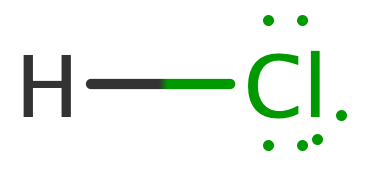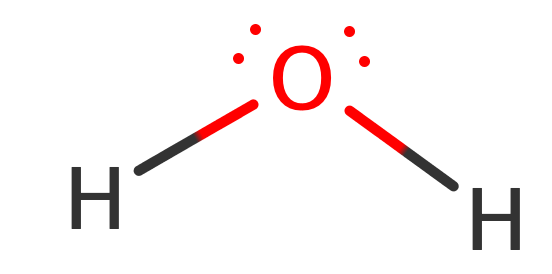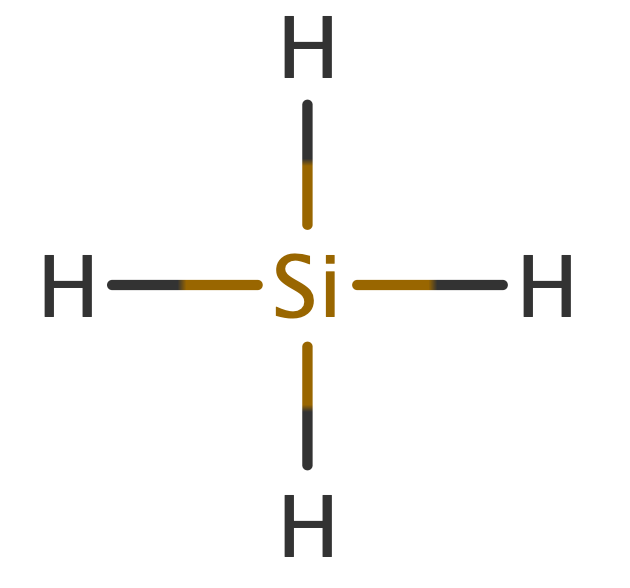# Problem: Arrange each of the following sets of compounds in order of increasing boiling point temperature:(a) HCl, H2O, SiH4

🤓 Based on our data, we think this question is relevant for Professor Yang's class at FSU.

###### FREE Expert Solution

HCl

total valence e- = 8 e-

Lewis structure:ΔEN = 3.0 – 2.2 = 0.8 polar covalent bond

dipole-dipole forces

H2O

total valence e- = 8 e-

Lewis Structure:ΔEN = 3.5 – 2.2 = 1.3 polar covalent bond

O connected to H hydrogen-bonding

SiH4

total valence e- = 8 e-

Lewis StructureΔEN = 2.2 – 1.8 = 0.4 polar covalent bond

no net dipole London dispersion forces###### Problem Details

Arrange each of the following sets of compounds in order of increasing boiling point temperature:

(a) HCl, H2O, SiH4